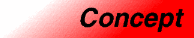UniqueAssociativeContainerCategory: containers Component type: concept

Description

A Unique Associative Container is an AssociativeContainer with the property that each key in the container is unique: no two elements in a Unique Associative Container have the same key.

Associated types

None, except for those defined by AssociativeContainer.

Notation

 X A type that is a model of Unique Associative Container a Object of type X t Object of type X::value_type k Object of type X::key_type p, q Object of type X::iterator

Valid expressions

In addition to the expressions defined in AssociativeContainer, the following expressions must be valid.

Name Expression Type requirements Return type
Range constructor
X(i, j)
X a(i, j);
i and j are InputIterator whose value type is convertible to T 
Insert element a.insert(t)   pair<X::iterator, bool>
Insert range a.insert(i, j) i and j are InputIterator whose value type is convertible to X::value_type.  void
Count a.count(k)   size_type

Expression semantics

Name Expression Precondition Semantics Postcondition
Range constructor
X(i, j)
X a(i, j);
[i,j) is a valid range. Creates an associative container that contains all of the elements in the range [i,j) that have unique keys. size() is less than or equal to the distance from i to j.
Insert element a.insert(t)   Inserts t into a if and only if a does not already contain an element whose key is the same as the key of t. The return value is a pair P. P.first is an iterator pointing to the element whose key is the same as the key of t. P.second is a bool: it is true if t was actually inserted into a, and false if t was not inserted into a, i.e. if a already contained an element with the same key as t. P.first is a dereferenceable iterator. *(P.first) has the same key as t. The size of a is incremented by 1 if and only if P.second is true.
Insert range a.insert(i, j) [i, j) is a valid range. Equivalent to a.insert(t) for each object t that is pointed to by an iterator in the range [i, j). Each element is inserted into a if and only if a does not already contain an element with the same key. The size of a is incremented by at most j - i.
Count a.count(k)   Returns the number of elements in a whose keys are the same as k. The return value is either 0 or 1.

Complexity guarantees

Average complexity for insert element is at most logarithmic.

Average complexity for insert range is at most O(N * log(size() + N)), where N is j - i.

Invariants

 Uniqueness No two elements have the same key. Equivalently, this means that for every object k of type key_type, a.count(k) returns either 0 or 1.